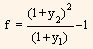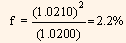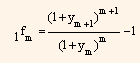## Deriving 6-month forward rates, Financial Management

Assignment Help:

Using details from table 8, let us compute the 6-month forward rate. Simple arbitrage principle, like the one used to compute the spot rates are used in this process.

For example, let us consider an investor who has 1-year investment horizon and has two alternatives, (i) to buy a 1-year T-bill or (ii) to buy a 6-month T-bill; and when it matures after six months to buy one more 6-month T-bill. If both investments have the same cash flows and carry the same risk, then they will have same value. The investor will be indifferent towards the two alternatives if both of them produce same returns in the 1-year investment horizon. But, to compare both the options, the investor needs to know the forward rate on the 6-month Treasury bill to calculate the yield available on a 6-month treasury bill that will be purchased six months from now. Using the 6-month and 1-year Treasury bill spot rate, the six month forward rate on a 6-month treasury bill can be equated.

Let us now see how to determine the forward rate. Assume that an investor purchases a six month T-bill for \$X. The value of the investment at the end of six months would be:

X (1 + y1)

Where,

y1 = One-half the Bond-Equivalent Yield (BEY) of the theoretical
6-month spot rate.

Let us say f represents one-half the forward rate on a 6-month treasury bill available six months from now. The future returns that the investor would be receiving on his investment one year from now if he reinvests his investment by purchasing a six month treasury bill at the end of first six months, would be:

X (1 + y1) (1 + f)

If we consider the alternative investment in 1-year T-bill and we assume that y2 represents one-half the BEY of the theoretical 1-year spot rate, then the total value of the investment at the end of one year would be:

X (1 + y2)2

We know that the investor will be indifferent towards the two alternatives if he receives the same return. We can represent it with the help of following equations,

X (1 + y1) (1 + f) = X (1 + y2)2

Solving for f,Now using the theoretical spot rates given in table 8, the 6-month and the 1-year
T-bill spot rate would be:

6-month bill spot rate = 0.0400, so y1 = \$1.0200.

1-year bill spot rate = 0.0420, so y2 = \$0.0210.

Substituting the values in equation (1) we get:So, the six month forward rate six months form now will be 4.4% (2.2% x 2) BEY. Now let us verify the values determined.  If \$X is invested in the 6-month T-Bill at 2% and the proceeds are then reinvested for six months at the 6-month forward rate of 2.2% then the total return form this option would be,

X(1.0200) x (1.0220) = 1.04244X

Now, if we invest the same amount i.e., \$X in 1-year T-bill at one half the
1-year rate, 1.02105%, then return from this option would be,

X (1.0210)2 = 1.04244 X

In a similar manner, 6 month forward rate beginning at any time period in the future can be calculated.  The notation we use to indicate 6-month forward rates is 1fm. In this, sub script 1 represents a 1-period (6-month) rate and subscript m represents the period beginning m period form now. When m is zero, then it represents current rate. Therefore, the first six months forward (1fm) rate is simply the current six month forward rate (y1).  The formula to determine a six month forward rate is:#### Leverage, what is the meaning of market feasibility? What are its different...

what is the meaning of market feasibility? What are its different types with their degree?

#### Capital gain or loss, An Investor can receive income from this ...

An Investor can receive income from this source when the bonds purchased at discount are held up to maturity or when he sells the bond before ma

#### Student, applicability of an operating cycle in vegetable growing in uganda...

applicability of an operating cycle in vegetable growing in uganda

#### Constructing index numbers - aggregates method, Aggregates Method Unde...

Aggregates Method Under the aggregates method of constructing an index number, we could have unweighted aggregates index and the weighted aggregates index. Unweighted Aggr

#### Partition of investment risk, Partition of Investment Risk The expecte...

Partition of Investment Risk The expected returns and the fluctuation in returns are two factors in evaluating investments. Expected Returns While the actual returns

#### What is institutional finance, What is Institutional Finance A natio...

What is Institutional Finance A nation's economic structure comprise a number offinancial institutions, like banks, pension funds, insurance companies, creditunions. These i

#### Criticism of walter’s model, (i) No External Financing: - Walter' model pre...

(i) No External Financing: - Walter' model presume that the firm's investment are financed exclusively by retained earnings and no external financing is used. If it was therefore t

#### Cost of equity from new common stock, Weaver Chocolate Co. expects to gain ...

Weaver Chocolate Co. expects to gain \$3.50 per share during the present year, its expected dividend payout ratio is 65%, its expected constant dividend growth rate is 6.0%, and its

#### University , After read all the available information carefully, prepare a ...

After read all the available information carefully, prepare a two page (double-spaced) essay and answer the following questions: Assume that we have the following data: C=100+0.50Y

#### What is nvp and cost of equity , 1. A standard arrangement for the orderly ...

1. A standard arrangement for the orderly retirement of long-term debt calls for the corporation to make regular payments into a(n):      A)  custodial account.     B)  sinking

### Write Your Message!#### Assured A++ Grade

Get guaranteed satisfaction & time on delivery in every assignment order you paid with us! We ensure premium quality solution document along with free turntin report!